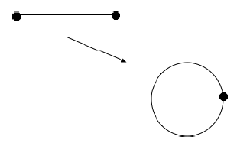# Contents

## Statement

###### Proposition

(quotient projections out of compact Hausdorff spaces are closed precisely if the codomain is Hausdorff)

Let

$\pi \;\colon\; (X, \tau_X) \longrightarrow (Y, \tau_Y)$

be a continuous function between topological spaces such that

1. $(X, \tau)$ is a compact Hausdorff topological space;

2. $\pi$ is a surjection and $\tau_Y$ is the corresponding quotient topology.

Then the following are equivalent

1. $(Y, \tau_Y)$ is itself a Hausdorff topological space;

2. $\pi$ is a closed map.

###### Proof

The implicaton $\left( (Y, \tau_Y)\, \text{Hausdorff} \right) \Rightarrow \left( \pi \, \text{closed} \right)$ follows since maps from compact spaces to Hausdorff spaces are closed. We need to show the converse.

Hence assume that $\pi$ is a closed map. We need to show that for every pair of distinct points $y_1 \neq y_2 \in Y$ there exist open neighbourhoods $U_{y_1}, U_{y_2} \in \tau_Y$ which are disjoint, $U_{y_1} \cap U_{y_2} = \emptyset$.

First notice that the singleton subsets $\{x\}, \{y\} \in Y$ are closed. This is because they are images of singleton subsets in $X$, by surjectivity of $f$, and because singletons in a Hausdorff space are closed, and because images under $f$ of closed subsets are closed, by the assumption that $f$ is a closed map.

It follows that the pre-images

$C_1 \coloneqq \pi^{-1}(\{y_1\}) \phantom{AA} C_2 \coloneqq \pi^{-1}(\{y_2\}) \,.$

are closed subsets of $X$.

Now since compact Hausdorff spaces are normal it follows that we may find disjoint open subset $U_1, U_2 \in \tau_X$ such that

$C_1 \subset U_1 \phantom{AAA} C_2 \subset U_2 \,.$

Moreover, by this lemma we may find these $U_i$ such that they are both saturated subsets. Therefore finally this lemma says that the images $\pi(U_i)$ are open in $(Y,\tau_Y)$. These are now clearly disjoint open neighbourhoods of $y_1$ and $y_2$.

## Applications

###### ExampleConsider the function

$\array{ [0,2\pi]/\sim &\longrightarrow& S^1 \subset \mathbb{R}^2 \\ t &\mapsto& (cos(t), sin(t)) }$
• from the quotient topological space of the closed interval by the equivalence relation which identifies the two endpoints

$(x \sim y) \;\Leftrightarrow\; \left( \left( x = y\right) \,\text{or}\, \left( \left( x \in \{0,2\pi\}\right) \,\text{and}\, \left( y\in \{0, 2\pi\} \right) \right) \right)$
• to the unit circle $S^1 = S_0(1) \subset \mathbb{R}^2$ regarded as a topological subspace of the 2-dimensional Euclidean space equipped with its

This is clearly a continuous function and a bijection on the underlying sets. Moreover, since continuous images of compact spaces are compact and since the closed interval $[0,1]$ is compact we also obtain another proof that the circle is compact.

Hence since continuous bijections from compact spaces to Hausdorff spaces are homeomorphisms the above map is in fact a homeomorphism

$[0,2\pi]/\sim \;\simeq\; S^1 \,.$

Last revised on May 28, 2017 at 14:08:48. See the history of this page for a list of all contributions to it.